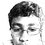# Am I Right?

I was going through "Challenging Mathematical Problems with Elementary Solutions (Vol 1)" by Yaglom & Yaglom.

I came across this problem,

Three points in the plane are given, not all on the same straight line. How many lines can be drawn which are equidistant from these points?

The solution states that if all three points are on the same side of the line, then they must lie on a parallel line, which is contradictory.

Thus, there are $3$ lines which exist such that $2$ points are on the same side. Thus, the book's answer is $3$.

However, when I solved I got $4$, a line perpendicular to the plane, through the centre of the circle, which passes through all the points.

Although the points are said to be in a plane, there was no constraint on the plane of the line.

Am I right? Or is it an accepted norm that lines are in the same plane as points?Note by Nanayaranaraknas Vahdam
6 years, 9 months ago

This discussion board is a place to discuss our Daily Challenges and the math and science related to those challenges. Explanations are more than just a solution — they should explain the steps and thinking strategies that you used to obtain the solution. Comments should further the discussion of math and science.

When posting on Brilliant:

• Use the emojis to react to an explanation, whether you're congratulating a job well done , or just really confused .
• Ask specific questions about the challenge or the steps in somebody's explanation. Well-posed questions can add a lot to the discussion, but posting "I don't understand!" doesn't help anyone.
• Try to contribute something new to the discussion, whether it is an extension, generalization or other idea related to the challenge.

MarkdownAppears as
*italics* or _italics_ italics
**bold** or __bold__ bold
- bulleted- list
• bulleted
• list
1. numbered2. list
1. numbered
2. list
Note: you must add a full line of space before and after lists for them to show up correctly
paragraph 1paragraph 2

paragraph 1

paragraph 2

[example link](https://brilliant.org)example link
> This is a quote
This is a quote
    # I indented these lines
# 4 spaces, and now they show
# up as a code block.

print "hello world"
# I indented these lines
# 4 spaces, and now they show
# up as a code block.

print "hello world"
MathAppears as
Remember to wrap math in $$ ... $$ or $ ... $ to ensure proper formatting.
2 \times 3 $2 \times 3$
2^{34} $2^{34}$
a_{i-1} $a_{i-1}$
\frac{2}{3} $\frac{2}{3}$
\sqrt{2} $\sqrt{2}$
\sum_{i=1}^3 $\sum_{i=1}^3$
\sin \theta $\sin \theta$
\boxed{123} $\boxed{123}$

Sort by:

The question assumes that you are working in the plane. Otherwise, it will say "three points in space".

Staff - 6 years, 9 months ago

Even if it is not specified that the lines have to be in the same plane, we have to assume this?

- 6 years, 9 months ago